Machine Learning Research
Volume 1, Issue 1, December 2016, Pages: 33-41

Marco Antonio Márquez-Vera1, *, Julo César Ramos-Fernández1, Blanca Diana Balderrama-Hernández2

1Department of Mechatronics, Politechnic University of Pachuca, Zempoala, Mexico

2Basic Education, Secretariat of Public Education, Pachuca, Mexico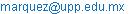(M. A. Márquez-Vera)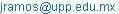(J. C. Ramos-Fernández)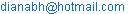(B. D. Balderrama-Hernández)

*Corresponding author

Marco Antonio Márquez-Vera, Julo César Ramos-Fernández, Blanca Diana Balderrama-Hernández. Adaptive Fuzzy Sliding Modes Observer for Phenol Biodegradation. Machine Learning Research. Vol. 1, No. 1, 2016, pp. 33-41. doi: 10.11648/j.mlr.20160101.14

Received: December 6, 2016; Accepted: January 6, 2017; Published: January 31, 2017

Abstract: There exist processes difficult to control as the chemical ones, in this work the bacterial grow rate in a biotechnological process is controlled, to make it, a fuzzy model was proposed, this control uses the clustering technique to improve the membership functions for the antecedents rules and least squares for the consequents; the control work in an acceptable manner, but in practice it is common to find that the actuators cannot respond to the signal control due saturation or its frequency response; so, a predictive control was used to anticipate the control signal. A comparative Table shows the comparison between different control horizons. Finally the use of a model reference can reduce the control signal amplitude and reduce some criterion errors.

Keywords: Fuzzy Logic, Observers Design Sliding Modes, Biodegradation

Contents

1. Introduction

The fuzzy control was applied in the seventies for systems that are structurally difficult to modeling, due its good performance, its popularity was increase and now a days it is common to find some machines where the word fuzzy is synonymous to high quality . In chemical process usually there are not inline sensors to know the states process, so it is difficult to design some control to have a tracking system , the knowledge of an expert use to be sufficient to establish the process in a determined set point to have stable state, but some problems can appear, first the process is nonlinear and often with poorly understood dynamics; second the model can be not well known, time variant and have a lot of uncertainties and unknown parameters, and finally to have states that cannot be measure inline by the lack of reliable sensors . All this problems can be affronted by using some modeled method  or by iterative learning [6,15,28]; and there is not a defined the time period, if it exits the measure cannot be done in the exact instant, it is expensive and techniques as centrifugation and spectroscopy must to be applied . So, the main idea in this work is to estimate the state process that cannot be measure inline, in this case, the process biodegrades phenol. It is important to note that the output process is neither known, working with chemical process has also the disadvantage of chemical reaction can be modeled as a non-stationary disturbance.

A fuzzy system is basically a rules-based system where the knowledge from an expert or an engineer can be used to build the rules base . As the system can be time variant and if the experience in working with chemical process is not enough, an adaptive system must to be implemented to estimate the outputs process.

Also the observer to be used must to be nonlinear, for stability it is proposed to use a fuzzy observer Sugeno type. An application of robust observers can be found in , where the idea was to monitor the algae cultivation. Now, the fuzzy logic had emerged as an alternative when there is vagueness in the process dynamic . For instance, a Sugeno inference system  is tuned because the rules consequents are linear submodels; one technique to have the local observers gains is using linear matrix inequalities [7,26], if local models are observable, an observer can be designed for each one such that all system is stable and with some decay rate. Also, artificial neural networks have been used to construct an observer as the shown by Lin  where dissolved oxygen concentration is controlled.

Recently, the type-2 fuzzy logic has appear as a new technique to make control laws , type-2 fuzzy logic is an extension of the nowadays called fuzzy logic type I where the fuzziness part or the second dimension is also fuzzy. However, there are some disadvantages and parameters design difficult to justify as the following :

There are a lot of parameters as the number of membership functions, the defuzzification method or the triangular norm to use that can be determined arbitrarily.

The rules base cannot have the optimal values or they have to change with time.

So, the fuzzy portion in a set is now also fuzzy, the uncertainties can be damping in a type-2 fuzzy system , and an upper type can be made, but for computational cost, only is used until type-2. To work with this kind of systems a type reducer should be implemented before the aggregation rules . And to optimize the membership functions there are some methods as the human evolutionary model .

In this work a fuzzy observer is implemented to measure the states process that cannot be obtained inline, the errors are computed to have an idea of the performance, the article is organized as follows: first the process description is shown, then some concepts about fuzzy logic are presented also the type-2 fuzzy logic; the next section shows the observer design with some concepts about sliding modes. Finally its application takes place with the conclusions.

The phenol is a common contaminant in water, some textile process and some substances to conserve wood has this substance , the biotechnological process takes the pollution as the feeding of microorganisms to purify the water, the activated sludge is commonly used  for waste water. The mathematical model was proposed and proved in , and an estimator design was simulated in . The mass balance equations for the states process are presented by the differential equations set (1), where the states are the biomass, phenol, intermediate and oxygen concentrations, and the last one is the volume inside the reactor, due agitation concentrations are assumed to be the same in all the reactor.

In this model, there exists a specific grow rate which was determined by a modification of the Monod and Haldane rates , it is computed as in (2), where each consumption rate are defined by (3) and (4), this grow rate can be also estimated with fuzzy logic as in  or the observer can be designed to estimate them as in .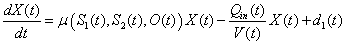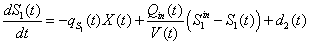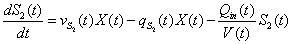(1)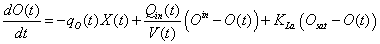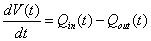where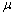is the biomass specific growth rate,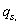,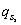and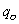are respectively, the specific consumption of phenol, metabolic intermediate and oxygen; and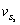; and is the specific production rate of metabolic intermediate.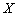,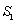,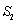, and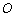are, respectively, biomass, phenol, intermediate and oxygen concentrations.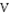is the volume liquid in the rector, the bacteria that consumes the phenol in this case is the pseudomonas putida. The different modes of culture can be directly coupled to these general settings: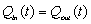in chemostat cultures,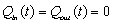in batch cultures and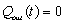in fedbatch cultures. Finally,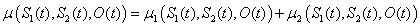and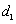are external disturbances.

The global specific growth rate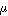is expressed as in (2):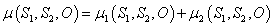(2)

where the terms of growth rate Haldane and Monod are modified equations respectively, and found by:(3)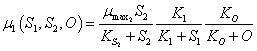(4)

The consumption constants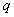in (1) are obtained with the growths rate as follows: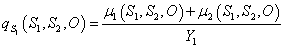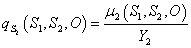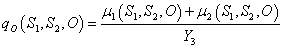The specific consumption rate of metabolic intermediate was linearly correlated to the specific growth rate of biomass on phenol as is shown: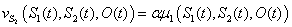In Table 1 are presented the values determined in modelling and they can be used for simulation, some of this parameters can be time variant, and it is one problem for indirect control because it is model dependent, so and adaptive algorithm must to be used in this case .

Table 1. Parameter values in phenol biodegradation process.

 Symbol Value Unit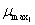0.04 h-1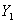0.67 mg mg-1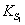2 mg l-1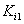17 mg l-1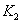91 mg l-1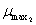0.30 h-1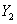0.75 mg mg-1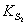75 mg l-1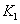66 mg l-1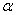6.70 mg l-1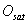30 mg l-1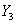0.90 mg mg-1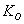0.40 mg l-1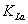24.61 h-1

In Table 2 is possible to appreciate the initial condition in the experiment, for simulation they can be used, as the process continues iteratively, some dead time must to be applied to have some similar initial conditions to the last iteration, some similar problem is shown in  for several iterations.

3. Fuzzy Logic

The fuzzy logic has emerged as an alternative to work with imprecisely or incomplete information, making decisions about processes that contain non-random uncertainty, such as the uncertainty in natural language, has been shown to be less than perfect . The notion of a fuzzy set provides a convenient point of departure for the construction of a conceptual framework, but more general than with ordinary sets. Essentially, such a framework provides a natural way of dealing with problems in which the source of imprecision is the absence of sharply defined criteria of class membership rather than the presence of random variables . And the idea is to work with linguistic variables easier to understand.

A fuzzy set is a set containing elements that have varying degrees of membership in the set; the elements of a fuzzy set are mapped to a universe of membership values using a membership function, so the elements now are ordered pairs. Similarly to classical operations sets as union, intersection and complement, there exist the algebra for fuzzy sets exists. There are some fuzzy membership forms which must to be convex , and all operators triangular-norm based can denote intersection between fuzzy sets .

Working with fuzzy sets makes possible to speak of fuzzy logic, where all logic operation can be expressed in fuzzy terms, it is an extension from the classical logic, and it is the mix of fuzzy sets and propositional logic . In classical logic, it is possible to get an inference result with some input information, such the cases in tollendo ponens and ponendotollens. A fuzzy logic proposition is a statement involving some concept without clearly defined function or boundary. Linguistic statements that tend to express subjective ideas and that can be interpreted slightly differently by various individuals typically involve fuzzy propositions.

Table 2. Initial condition process.

 Symbol Value Initial biomass (mg l-1) 4500 Initial phenol (mg l-1) 250 Initial oxygen (mg l-1) 28 Initial intermediate (mg l-1) 0 Operation mode Fedbatch Feeding phenol concentration (mg l-1) 1020

3.1. Fuzzy Inference Systems

As it is possible to work with linguistic variables in fuzzy logic, the knowledge form an expert who knows how to model or control a process can be used to construct an inference machine, the fuzzy logic can represent ideas and the way as a person think and take a decision; it is a part of artificial intelligence. Once some variables are fuzzified (a membership value with respect to a set is assigned to each value in the discussion universe) some rules can be built and they are used to take the decision as output system.

The main inference systems in fuzzy logic are the Mamdani and Sugeno types; in the first one, the inputs or antecedents rules and the output or consequents rules are fuzzy, then a defuzzification should be done . In this work, the Sugeno inference system is used because its consequents are linear functions of his inputs. Some techniques are implemented to synthesize the system; it is useful when it is difficult to make a mathematic model, or when the system is not well understood. The fuzzy rules have the following presentation: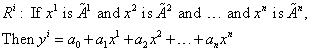where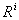is the i-th rule,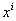is the vector input to fuzzify,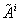is a fuzzy set,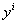is the output rule and the right side in the consequent is a linear combination from inputs. A rule set can be founded to express in some linguistic way, a process model or a control action, the knowledge from an expert can be used to fill the rules base, or made by an algorithm as least squares or clustering . The rules base is a synthesis of the rules and also called the fuzzy associative memory as shown in Table 3, the disadvantage is that only two variables can be used, so different associative memories must be done; in control theory, the Table 3 can express the control law as output in the matrix elements, where the rows and columns can represent a fuzzy partition , of error and its derivative, in this case, a fuzzy PD control is formed.

Where the words Big Negative, Negative, Zero, etc. are the linguistic variables and they are represented by a membership function, in Table 3 are shown five linguistic variables for each input, it means that there were a fuzzy partition with five membership functions as in Fig. 1, where five triangular membership functions are the fuzzy partition of universe x, as it is not easy to determine always all the universe, semi-trapezoid functions can be used as the first and the last membership functions.

To have the output from rules base, an aggregation rules is done, similar to defuzzification in Mamdani case, but it is faster because in this step, a crisp value is obtained in (5) it is possible to appreciate this concept. To have an observer states, the consequents rules were defines as local state space models, the output can be seen as an interpolation between all consequents, linear systems in this case, and it also works as a filter, the question now is how to design an stable observer because the stability of all local submodel does not implies global stability  so a common Lyapunov function must to be found for all local submodels .

Table 3. PD control fuzzy associative memory.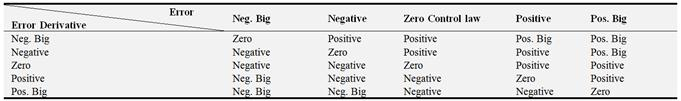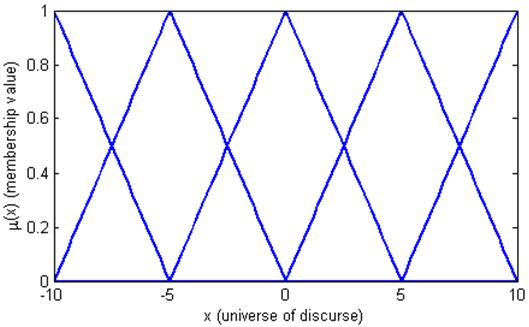Figure 1. Fuzzy partition in five linguistic variables of variable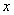.(5)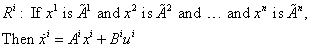3.2. Type-2 Fuzzy Logic

The membership function can have different presentation, the Triangular, Trapezoid and Gaussian types are the common, the triangular ones are useful for its computational cost, they are easy to program, the Gaussian type are smooth functions, so gradient descendent method can be improved in algorithms . The number of fuzzy partitions and the shape of membership functions can be arbitrarily chosen, to fix this situation, a generalization can be done, it is the type-2 fuzzy logic, where the membership is also fuzzy, now there are three dimensions, and a representation is shown in Fig. 2 where two dimensions are defined between 0 and 1 because they are membership values, to work with this kind of functions can take some time to compute, so it is possible to work with its bounds, in Fig. 3 it is presented two membership functions that can represent the limits and they are called the foot of print, as there are two membership degrees a type reducer is needed , for simplicity, a higher dimension can be used but it is just worked until type-2  for computational cost.

The type-2 fuzzy sets enable modeling and minimizing the effects of uncertainties in the fuzzy systems. Unfortunately, type-2 fuzzy logic is more difficult that the type-1. As a justification there are some uncertainties not considered in the type-1 .

The mining of the words that are used in the fuzzy rules can be uncertain.

Consequents may have histogram of values associated with them.

Measured variables to be fuzzified can be noisy.

To work with type-2 fuzzy logic it is proposed to see membership functions as in Fig. 3, where there are two limits in the membership, they are called the upper and lower membership functions, with this idea it is possible to vary some parameters as the width or the center of functions and in this way have the limits, in  is proposed to get the average of memberships between limits to make the type reducer, by using this idea, the observer design was made an compared with the type-1 fuzzy observer.

If a fuzzy partition is made to get a fuzzy submodels, there will be a big fuzzy associative memory and in three dimensions, in the case of selecting three membership functions for each state, there will be 243 fuzzy rules, in simulation a good performance can appear, but in practice is not easy to make, fortunately the bioprocess presents slow dynamics and the computational cost seems not to be critical. However a cascade model  can be built an to have around 100 rules with three membership functions in each state, if a common matrix can be find, it is possible to have global stability in the observer, finally with states estimated to make a feedback states.

Finally, type-2 fuzzy sets can be used to convey the uncertainties in membership functions of type-1 fuzzy sets, due to the dependence of the membership functions on available linguistic and numerical information. For a type-2 Sugeno inference system it is not needed a type reduction because the rules consequents are not fuzzy. In next section, it will be implemented observers for concentrations based in type-1 and type-2 fuzzy logic, the observer gain is fuzzy and they are used nine rules for each observer.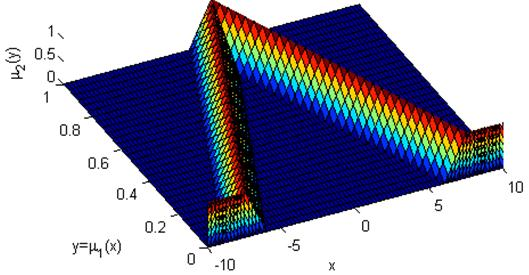Figure 2. Type-2 Membership function.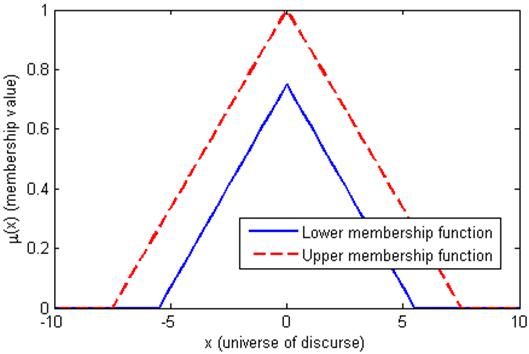Figure 3. Type-2 Foot of print from Fig. 2.

4. Sliding Modes

The sliding modes were proposed to make a control law that cannot be model dependent , so it is a direct control. The sliding modes are formed by two control actions, one is called the equivalent control, it can be built by the model process or by using the proposed bounds for the model, it can be fuzzily approach , the other control action is the switching control, which depends if the error or some function from error, called the sliding surface, is positive or not, in this way, it is a discontinuous control, that has an effect of chattering in the output system, this can be eliminated by using a fuzzy PI for example , in (6) it is possible to see the control law with sliding modes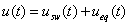(6)

where usw(t) is the switching control and ueq(t) is the equivalent control, by using the model structure (7), each control action is defined next,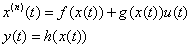(7)(8)

where, r(t) denotes the reference signal and (n) means its n-th derivative, the system must to be control affine, so using the dilution rate D(t)=Qin(t)/V(t)  the model process is as (7) being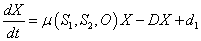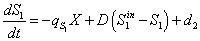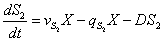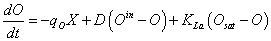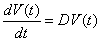Finally, the sum is the sliding surface which can be presented as a polynomial that should be stable  as is shown in (9), in this work it was used only the error without derivatives as sliding surface.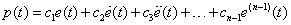(9)

The gain η is fuzzy and can be time variant depending from input signals, as the magnitude of signals are different each observer must to be tuned. An adaptive method is used to make this, some similar method makes fuzzy the sliding surface p(t) as in .

5. Fuzzy Sliding Modes Observer

A states observer is a copy of the control system that estimates the state variables with the input and output measurements , it is possible to make it if the variable to estimate is observable, so, it is important to get observability at least for unknown variables [18, 28], some concentrations in this case.

The observer design was implemented for three of the five system’s states, so there were made three observes of full rank, the states that were measured inline were the volume and the oxygen concentration, and the states to approach are the biomass, the phenol and the metabolic intermediate concentrations. To have the observers sliding modes based, the signals are approached by using (10).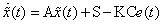(10)

where A is a common matrix that represents a stable and observable system, Sis the switching control that is defined in (11), K is a gain to improve the convergence rate, C=] is the output matrix and e(t) represents the error, for each observer the first term for error are the biomass, the phenol or the metabolic intermediate concentration denoted for example z(t), and the others are the states measured O(t) and V(t). Being A the next matrix.The switching control has a matrix P that is proposed for Lyapunov stability, and the sign function of error can produce the chattering so a gain η is used.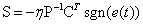(11)

The matrix P was proposed to be symmetric positive definite from Lyapunov’s equation (12) and if the P matrix is common to all observers, the system is asymptotically stable , almost for the universe of discourse proposed for the measured variables .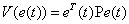(12)6. Fuzzy Observers for Concentrations

The phenol biodegradation process is worked in fedbatch mode, which implies a cycle where a feeding stage takes some time and a feeding profile describes the inlet which is shown in Fig. 4, then a dead time where only agitation and aeration take place is when the biomass makes the consumption of phenol, finally the decantation is done where there are not agitation, finally the water without pollution is removed from the upper place due the microorganisms make an aggregation and deposited at the bottom of reactor . This cycle is done continuously and water purification is the result, however, an increase in biomass concentration is possible, it is common to make a purge and incinerate the biomass that is not needed. In this work the control action was not applied, so phenol consumption is small, the observers design is useful for make control actions to purify water and to prevent biomass die for a high toxic influent. An exponential function for define the inlet profile can improve the bioprocess .

The use of fuzzy logic was to find the gain for observers, in this case three observers was implemented to estimate the concentrations, it is important because the output system (phenol concentration) is not measured, once the input systems are fuzzyfied, nine rules are evaluated to have the gains, the results are compared with the outline measures, the sampling time was of 15 minutes due it is difficult to take sampling quickly and because the process dynamics are, fortunately, slow. An observer was design in  where the biomass concentration is estimated. In Fig. 5 it is possible to see a scheme for the observers, where the inputs are the error estimation and the fuzzy values for oxygen concentration and the volume, then by applying a fuzzy gain η and the sliding modes, the estimated concentrations are found. In Fig. 6 it is possible to see the biomass estimation, the error is small, in Fig. 7 and Fig. 8, the estimates concentrations for phenol and the intermediate are shown, the sampling period was of 15, type-2 fuzzy logic was used in order to improve observers, to have an idea for errors dimension, the ISE was applied, the ITAE presents small values due convergence and as the initial values are far from real values, at the beginning the time is small and the error magnitude is not too important. Finally some relative error is presented due the concentrations values are different and absolute error loses importance.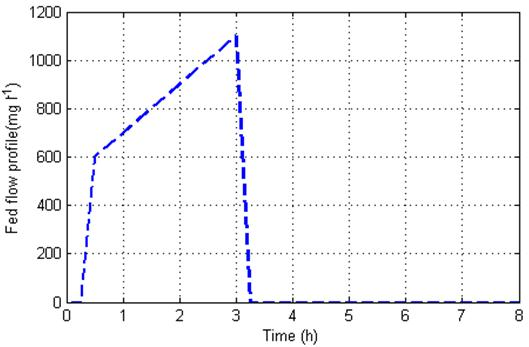Figure 4. Feed flow rate profile.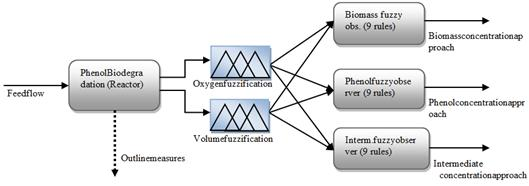Figure 5. Fuzzy observers scheme.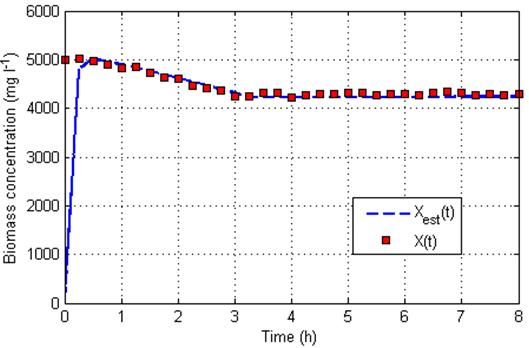Figure 6. Biomass estimation.

The ISE is the integral of square error (13) , and in Table 4 the estimate errors are presented, and also the ITAE (14) is used to have an idea about the observer performance.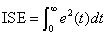(13)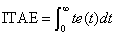(14)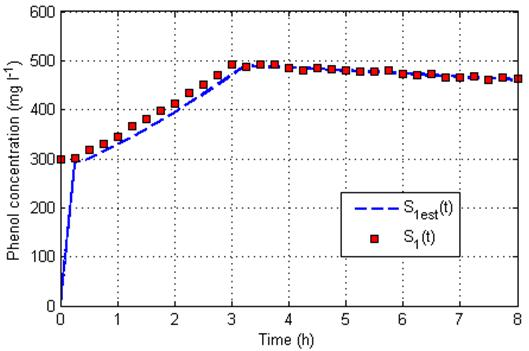Figure 7. Phenol estimation.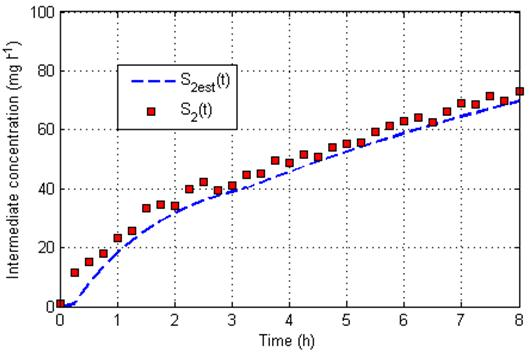Figure 8. Intermediate concentration estimation.

Table 4. States estimating errors.

 State estimating ISE ITAE/max(E) ISE/max(E) Biomass concentration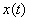2.5008e7 11.4521 2.4506e5 Phenol concentration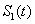9.2221e4 1.7025 911.6582 Intermediate concentration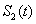166.2725 1.3223 1.6425

Something interesting is that, as complete states observers are designed, all states are approach, fortunately, volume and oxygen concentrations are known and used to estimate the concentrations process, but if it is compared the real values measured and its estimates, a big error takes place in the oxygen case, it can be modified by changing the matrix A in the observer design, but not well results were found, Fig. 9 shows the volume estimate, and no problem is presented, but in Fig. 10 is shown the oxygen estimate, it is possible to see that observer is stable but a big error is obtained, the lines are the estimates values for each observer, there are three of them, one for each concentration; for the volume, all the observers give the same value and it is near to the real value. Different values in matrix A which is defined to have observability in the system only changes the convergence rate but not a good performance in this state, in the same way, different values are obtained from observers for the oxygen concentrations and no one has a good performance.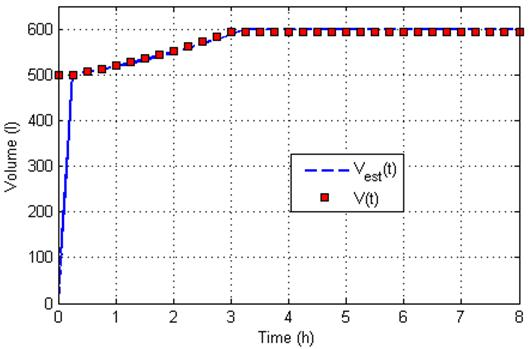Figure 9. Volume estimation.

]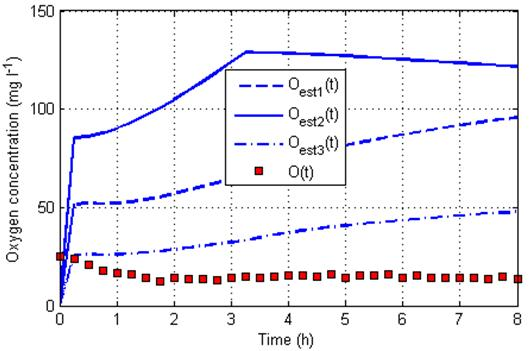Figure 10. Oxygen concentration estimation.

It is an alternative to get the states values and to make a control action  to increase the phenol consumption. The idea of averaging the membership given for the upper and lower functions was used  to simplify the fuzzy system. Table 4 shows that with the ITAE the errors are small at the end of the process and they can be used to have bioprocess instrumentation.

7. Conclusions

The fuzzy observers are a method to make nonlinear observers, when the process is not well known, some criterions can be used, an alternative is the sliding mode observers, but to know the cotes model to put the gains is not too easy, they were made fuzzy to improve the performance. The fuzzy logic can filter and eliminates some uncertainty in the parameters model and also in the dynamics model by filtering the noise. In this work was attacked a frequent problem in chemistry and biotechnology, for control issues to know all states in the process is a good idea to modify the dynamic and have a regulator, or to know the output at least to have the closed loop control. As there are not inline sensors, control actions are not possible to make in time, the iterative learning is an alternative.

It was possible to see that with information of inline measures from oxygen and volume, it was sufficient to approach the other variables and also the variable to control in experiment and to have a feedback states. To reduce the computational cost, it was proposed to use triangular membership functions and only three of them in the fuzzy inference system (Sugeno type), and the errors are in Table 4. In experiments it was found that the intermediate generated is also toxic, so it is important to know its concentration, it was used type-2 fuzzy logic, to have two membership functions, the lower and upper functions were used and were tuned with the human evolutionary model.

This technique can reduce the time, work and cost to have measures from an experiment for the reason of to have a near idea of the real values in states. The closed loop is in time and not only for iterations. Errors from noise can be reduced and as future work it is proposed to have an adaptive fuzzy model to get the equivalent control, and disturbance can be applied to verify the model by treating not to eliminate the biomass due it is an acclimated activated sludge.

Finally, the estimation of the oxygen concentration is different in each observer, but its value does not converge to the real one, fortunately it is a measure that is known and it is stable.

Acknowledgements

The authors wish to thank to Chemistry Research Center of the Autonomous University of the Hidalgo State for its collaboration in experiments and results, and for its feedback knowledge and participation, especially to Dr. Gabriela Vázquez.

References

1. A. Aceves, Usos y Abusos de la Lógica Difusa para el Control de Procesos: Una Alternativa para Modelar lo Incompleto de la Información y lo Impreciso de una Observación, Proc. Conf. Of Con Mantenimiento Productivo, Mexico. (2001) pp. 12-17.
2. K. Astrom, B. Wittenmark, Adaptive Control. Pearson Education. India, 2006.
3. K. Astrom, T. Hagglund, Advanced PID Control. Instrumentation, Systems, and Automation Society. United States of America, 2006.
4. R. Babuska, Fuzzy Modeling for Control. In Dr. Hans-Jurgen Zimmermann (Ed). International Series in Intelligemt Technologies, Aachen, Germany, 1998.
5. O. Bernard, G. Sallet, A. Sciandra,Nonlinear Observers for a class of Biological Systems: Applications to Validation of a Phytoplanktonic Growth Model. IEEE Transactions on Automatic Control, 43 (8) (1998) 1056-1065.
6. Z. Bien and J. Xu, Iterative Learning Control: Analysis, Design, Integration and Applications. Kluwer Academic Publishers, Massachusetts, 2008.
7. S. Boyd, L. El Ghaoui, E. Feron, V. Balakrishnan, Linear Matrix Inequalities in System and Control Theory. Society for Industrial ans Applied Mathematics. Philadelphia, 1994.
8. O. Castillo, P. Melin, Type-2 Fuzzy Logic: Theory and Applications. Studies in Fuzzyness and Soft Computing 223, Springer, 2008.
9. I. Dunn, E. Heinzle, J. Ingham, J. Prenosil, Biological Reaction Engineering. Wiley-VCH Verlag GmbH, Germany. 2003.
10. C. Feudjio. P. Bogaerts, J. S. Deschenes, A. V. Wouwer, Design of a robust Lipschitz observer-application to monitoring of culture of micro-algae Scenesdesmus obliquus. IFAC-PapersOnLine. 49(7) (2016) 1056-1061.
11. J. M. Guadayol. Control, Instrumentación y Automatización de Procesos Químicos: Problemas. Ediciones de la Universidad Politécnica de Cataluña, Barcelona, 1999.
12. Q. P. Ha, Q. H. Nguyen, D. C. Rye, Fuzzy Sliding-Mode Controllers with Applications. IEEE Transactions on Industrial Electronics, 48 (1) (2001) 38-46.
13. N. Karnik, J. Mendel, Q. Liang, Type-2 Fuzzy Logic Systems, IEEE Transactions on Fuzzy Systems. 7 (6) (1999) 643-658.
14. B. Kuo. Sistemas de Control Digital. Compañía Editorial Continental. Mexico, 2003.
15. Z. Lendek, T. Guerra, R. Babuska, B. De Schutter.Stability Analysis and Nonlinear Observer Design Using Takagi-Sugeno Fuzzy Models. Studies in Fuzziness and Soft Computing. Springer. India, 2010.
16. M. J. Lin, F. Luo, Adaptive neural control of the dissolved oxygen concentration in WWTPs based on disturbance observer. Neurocomputing. 185 (2016) 133-141.
17. R. Marino, P. Tomei, Adaptive Observers with Arbitrary Exponential Rate of Convergence for Nonlinear Systems. IEEE Transactions on Automatic Control. 40 (7) (1995) 1300-1304.
18. M. A. Márquez, C. Ben-Youssef, G. Vázquez, J. Waissman, Iterative Learning Control of a SBR Reactor by using a Limited Number of Samples, Proc. Conf. of 2nd International Meeting on Environmental Biotechnology and Engineering, D.F. Mexico 2006 pp. 81-89.
19. M. A. Márquez, J. Waissman, O.Gutú, Fuzzy Model Based Iterative Learning Control for Phenol Biodegradation. P. Melin et al. (Eds), Foundations on Fuzzy Logic and Soft Computing, Lecture Notes in Artificial Intelligence 4529, 2007, pp. 328-337.
20. J. M. Mendel, R. I. John, Liu, Interval Type-2 Fuzzy logic Systems Made Simple, IEEE Transactions on Fuzzy systems. 14 (6) (2006) 535-550.
21. O. Montiel, O. Castillo, P. Melin, R. Sepúlveda.Improving the Human Evolutionary Model: An Intelligent Optimization Method. International Mathematical Forum. 2 (1) (2007) 21-44.
22. F. Muñoz, C. Ben-Youssef, Biomass and Phenol Estimation using Dissolved Oxygen Measurement, Proc. Conf. of Electronics, Robotics and Automotive Mechanics Conference, Cuernavaca, Mexico, 2006.
23. H. Nguyen, N. Prasad, C. Walker, E. Walker, A First Course in Fuzzy and Neural Control. Chapman & Hall/CRC. Florida, 2003.
24. S. Nuñez, H. De Battista, F. Garelli, A. Vignoni, J. Picó, Second-order sliding mode observer for multiple kinetic rates estimation in bioprocesses. Control Engineering Practice. 21 (2013) 1259-1265.
25. S. Nuñez, F. Garelli, H. De Battista, Product-based sliding mode observer for biomass and rate estimation in Luedeking-Piret like processes. Chemical Engineering Research and Design. 105 (2016) 24-30.
26. K. Passino, S. Yurkovich, Fuzzy Control. Addison Wesley Longman Inc. California, 1998.
27. T. Ross, Fuzzy Logic with Engineering Applications, John Wiley & Sons, Ltd, West Sussex, 2008.
28. A. Tayebi, J. Xu, Observer-Based Iterative Learning Control for a Class of Time-Varying Nonlinear Systems, IEEE Transactions on Circuits and Systems, 50 (3) (2003) 452-455.
29. M. Teixeira, E. Assunçao, R. Avellar,On Relaxed LMI-Based Designs for Fuzzy Regulators and Fuzzy Observers. IEEE Transactions on Fuzzy Systems, 11 (5) (2003) 613-623.
30. D. Theilliol, J. C. Ponsart, J. Harmand, C. Join, P. Gras,On-line Estimator of unmeasured Inputs for Anaerobic Wastewater Treatment Process. Control Engineering Practice, 11 (2003) 1007-1019.
31. G. Vázquez, C. Ben-Youssef, J. Waissman, Two Step Modelling of the Biodegradation of Phenol by an Acclimated Activated Sludge, Chemical Engineering Jornal 117 (3) (2006) 245-252.
32. W. Y. Wang, M. L. Chan, C. C. James, T. T. Lee, H∞ Tracking-Based Sliding Mode Control for Uncertain Nonlinear Systems via and Adaptive Fuzzy-Neural Approach. IEEE Transactions on Systems, Man and Cybernetics, Part B: Cybernetics, 32 (4) (2002) 483-492.

 Contents 1. 2. 3. 3.1. 3.2. 4. 5. 6. 7.
Article ToolsAbstractPDF(323K)